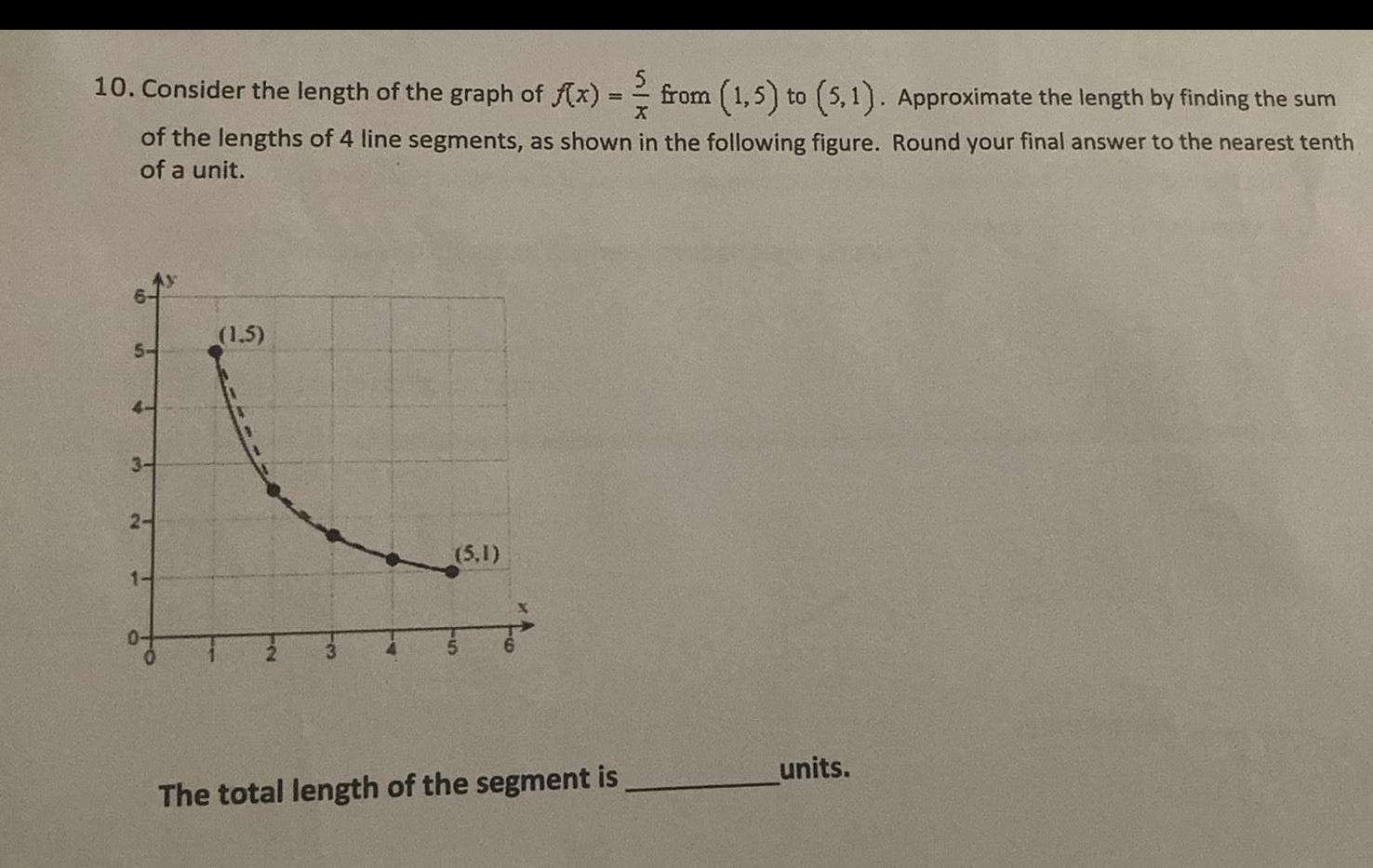Question:

# 10. Consider the length of the graph of f(x) = == from (1,5)

Last updated: 8/12/202210. Consider the length of the graph of f(x) = == from (1,5) to (5,1). Approximate the length by finding the sum X of the lengths of 4 line segments, as shown in the following figure. Round your final answer to the nearest tenth of a unit. 5- 4- 3- 2- 1- (1.5) 12 (5,1) The total length of the segment is units.